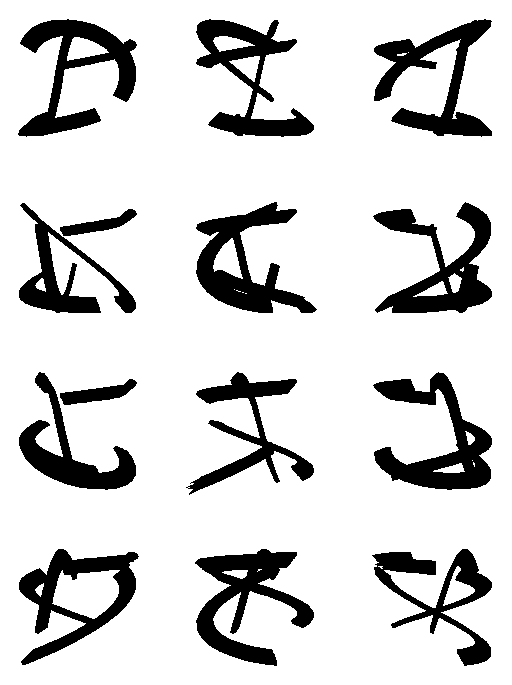# Environment for creative processing of text and numerical data## Picture writing

S.Mustonen (1990)### Edit field:

```   1 *
2 *`PLOT X(T)=M-0.5+R*cos(H1*(M+9)*T/5),`
3 *     `Y(T)=N-0.5+R*sin(H2*(N+9)*T/5)`
4 *M=1,3,1 N=1,4,1 h=4,10,2 H1=6*sin(3.7*h) H2=5*cos(5.8*h)
5 *R=0.25,0.32,0.01 XSCALE=0,4 YSCALE=0,4 DEVICE=PS,EX.PS
6 *HEADER= FRAME=0 SIZE=1309,1300 XDIV=0,1,0 YDIV=0,1,0
7 *T=[line_width(2)],1.57,1.87,0.02
8 *

```